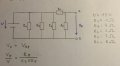# Thévenin Theorem - Problem with equivalent voltage & resistance

#### tarikgr

Joined Feb 29, 2016
3
Hey Guys!

I study mechanical engineering in Germany and somehow I got a problem with calculating with the Thévenin Theorem. I'll write an exam in 5 days so your help is much appreciated!!
I've read the articles on this side but somehow I still get wrong results.
In nearly every task we have to calculate the open-circuit voltage.
So my guessing was:
The open-circuit voltage behaves the same to the resistor it drops of at, as the voltage sorce to the resistors it drops of at. (sorry if I'm translating wrong, but hopefully you'll get my point. I've also posted a picture with the Task and the equations I used.).
Apparently my calculation is wrong. You'll probably see that I don't understand this subject that well but hopefully you'll see what my comprehensive issues are and maybe you can help me.THANK YOU!

#### Dodgydave

Joined Jun 22, 2012
10,592
If the voltage supply is 15V and the resistors are correct values, then vout = R8/R7+R8 x V = 8V.

#### crutschow

Joined Mar 14, 2008
31,156
The output voltage Vo/V = R8/ (R7 +R8), not R8 / (R7 || R8).
R7 || R8 is the Thevenin equivalent output resistance, which is unrelated to the open circuit output voltage.
Your equation would give Vo greater than V which obviously is impossible.

To derive Vo you can calculate the current through R7 and R8 as I = V / (R7 + R8).
The Vo voltage drop across R8 (from Ohm's law V = IR) is then Vo = V / (R7+R8) * R8 = V*R8 / (R7 + R8) or
Vo / V = R8 / (R7 + R8), as above.

Incidentally this should be in the Homework forum, not the Projects forum.

#### tarikgr

Joined Feb 29, 2016
3
Thank you guys!
R7 || R8 is the Thevenin equivalent output resistance, which is unrelated to the open circuit output voltage.
I don't see why this is the case. I get that the resistor of V has to be greater than the one of Vo but if you look at the Circuit, R6 and R7 are in parallel, and as I see it the complete Voltage V drops through R6 and R7.

I know that my thoughts may be a bit stupid, but this is my first time learning anything about electric engineering and I really want to understand it so I hope you guys have a bit patience. Thank you very much!!

#### Dodgydave

Joined Jun 22, 2012
10,592
NO, resistors R1,4,6 are in parallel, R7,8 are in series.

#### AnalogKid

Joined Aug 1, 2013
10,178
It is a trick question. Assuming a theoretically perfect battery that makes a perfect 15 V no matter what the load current is, then R1, R4, and R6 are not relevant to the problem. The two battery connections go straight to R7 and R8 in series. If you cut out R1, R4, and R6 one at a time, the voltage across R7 and R8 is a steady 15 V. So for this particular question, the entire circuit is 15 V across a total of 15 ohms. This makes the Ohm's Law arithmetic pretty simple.

ak

#### tarikgr

Joined Feb 29, 2016
3
NO, resistors R1,4,6 are in parallel, R7,8 are in series.
I don't know if I didn't understand this correctly or maybe I didn't have drawn it accurate, but there is a node between R7 and R8 (in the original homework), and I thought that if they're separated by a node and are angled like that, it is like a parallel circuit.

Am I wrong?

#### AnalogKid

Joined Aug 1, 2013
10,178
Yup. If two resistors are connected together at one end but not at the other, that is a series connection. If they are connected together at both ends and look like parallel lines, that is called a ... wait for it ... p.a.r.a.l.l.e.l connection.

https://en.wikipedia.org/wiki/Series_and_parallel_circuits

ak

#### crutschow

Joined Mar 14, 2008
31,156
I don't know if I didn't understand this correctly or maybe I didn't have drawn it accurate, but there is a node between R7 and R8 (in the original homework), and I thought that if they're separated by a node and are angled like that, it is like a parallel circuit.

Am I wrong?
Yes.
Why would the angle at which the resistors are drawn on a schematic have any bearing on whether they were serial or parallel?#### ErnieM

Joined Apr 24, 2011
8,346
Parallel and series can be funny to see. Takes lots of training to spot them right away.

Start at V: everything that connects to The top and bottom of it is in parallel. That means R1, r4, and r6. R7 and r8 are in series, and that series pair is also in parallel with V.

R1,4,6 do not affect the series pair r7,8 so they may be ignored to compute the open circuit voltage. Seems you saw the voltage divider and are on the way to computing it correctly at 8 volts.

Next comes the thev resistance. That you get by shorting the voltage source. That makes r1,4,and 6 go away in the short, and you are left with r7 and 8 now in parallel. You get a numbe close to 3 3/4 th ohms.

So sometimes r7 and r8 are in series, sometimes parallel. It depends on how you are looking at them.

#### WBahn

Joined Mar 31, 2012
27,946
MOD NOTE: Thread moved from forum The Projects Forum